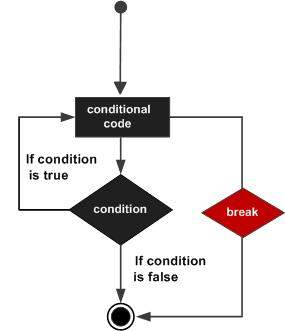# C++ break statement

The break statement has the following two usages in C++ −

• When the break statement is encountered inside a loop, the loop is immediately terminated and program control resumes at the next statement following the loop.

• It can be used to terminate a case in the switch statement (covered in the next chapter).

If you are using nested loops (i.e., one loop inside another loop), the break statement will stop the execution of the innermost loop and start executing the next line of code after the block.

## Syntax

The syntax of a break statement in C++ is −

```break;
```

## Flow Diagram## Example

```#include <iostream>
using namespace std;

int main () {
// Local variable declaration:
int a = 10;

// do loop execution
do {
cout << "value of a: " << a << endl;
a = a + 1;
if( a > 15) {
// terminate the loop
break;
}
} while( a < 20 );

return 0;
}
```

When the above code is compiled and executed, it produces the following result −

```value of a: 10
value of a: 11
value of a: 12
value of a: 13
value of a: 14
value of a: 15
```
cpp_loop_types.htm